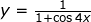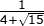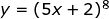## Posts

Showing posts from June, 2010

### Differentiation of Trig functions Part IIDifferentiation of trig functions using Chain rule, Product rule and Quotient rule Example 1 Find Technique: Use of Chain rule Example 2 Given that , show that Technique: use Quotient Rule What's next ? Check out other topics in Contents Page Revise the basics of the differentiation of trig functions

### Differentiation and Integration of Trigonometric functionsA lot of students confuse the differentiation of trig functions with the integration of trig functions The most effective way is to study them together Remember that: We can differentiate tan x but we cannot integrate tan x Example 1 Find Example 2 Note that Example 3 Find Example 4 Note that

### Surds, Log and IndicesAn interesting question showing these 3 topics can be tested in a single question Question Express as i) Hence show that ii) Given that , prove that Techniques tested Rationalization Indices: Power Law: Answer Technique used: Rationalization i) Techniques used: Indices : Power Law : From LHS ii) This part of the question requires us to use the Substitution technique Using the quadratic formula, Recall that therefore Technique used: Taking log on both sides Recall that in part (i) we showed that Interesting question, dont you think ? For more interesting questions subscribe to my blog by entering your email address at the top right hand corner Have an interesting question to share? send me an email at yapye at (@) yahoo dot com dot sg For a revision of surds, click here For a revision of logarithms, click here For a revision of indices, click here

### Integration Techniques Part II"Anti Chain Rule" This isnt an official maths term. Just something to help students remember how this technique is applied Recall the use of chain rule in differentiation Differentiate with respect to x Chain rule : "Bring down power, negate power by one multiply by the differentiation of the terms within the brackets" Integration using "Anti Chain rule": Increase power by one, divided by new power and the differentiation of the terms within the brackets" Question 1 Note that there are some limitations to "anti chain rule" You cannot . "Anti chain rule" is ony possible with linear factors Question 2 Integration as anti differentiation In question 1, when we differentiate y, we get When we integrate , we get back Question 3 Given that a) Find b) Hence evaluate b)

### Integration techniques Part IIntegrating term by term Formula: where c is a constant Example 1 Example 2 Example 3 Example 4